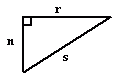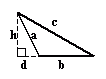Email us to get an instant 20% discount on highly effective K-12 Math & English kwizNET Programs!

#### Online Quiz (WorksheetABCD)

Questions Per Quiz = 2 4 6 8 10

### MEAP Preparation - Grade 5 Mathematics3.3 Classifying Triangles by Angles

#### Classification of triangles based on angles

1. Acute angled triangle:

• If all the angles of a triangle are acute angles, then it is called an acute angled triangle.

•2. Right angled triangle:
• A triangle in which one of the angles is a right angle is called a right angled triangle.

• In a right angled triangle, the side opposite to the right angle is called its hypotenuse.

•3. Obtuse angled triangle:
• A triangle containing an obtuse angle is called an obtuse angled triangle.

•Directions: Read the above properties of triangles and answer the following questions:
1. Illustrate each of the above properties by drawing a triangle.
2. Write in your own words, how to classify triangles according to their angles.

 Q 1: If the angles of a triangle are given by 100 degrees, 30 degrees and 50 degrees, then the triangle is an right angled triangle.TrueFalse Question 2: This question is available to subscribers only!